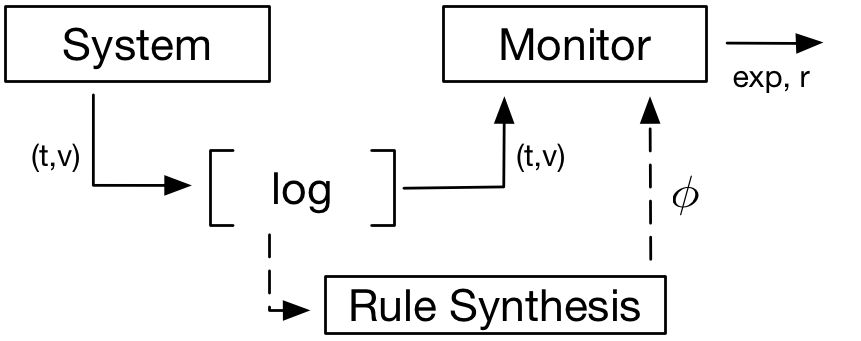# Monitoring with STL

In this project, our goal is to represent labeled events as temporal logic formulas. For this purpose, we develop fully automated algorithms, and implement them in academic tools. The formulas generated by the tools are can be used as monitoring rules. In addition to the synthesis of monitoring rules, the formula synthesis algorithms are used to generate the causes of the labeled events.

# Online Monitoring

In online monitoring, it is crucial to detect a deviation from normal behavior as soon as it occurs.  During online monitoring, the system traces are checked against monitoring rules in real-time to detect such deviations. In general, the rules are defined as boundary conditions by the experts of the monitored system. In this project, we represent monitoring rules in past time Signal Temporal Logic (ptSTL), develop novel methods to 1) synthesize such formulas from labeled data 2) perform online monitoring efficiently.System monitoring. Solid lines represent the real-time data flow, whereas the dashed lines represent the offline rule synthesis. ptSTL formula phi is a monitoring rule and r is the monitoring output.

## Efficient online monitoring from ptSTL formulas

Efficient evaluation algorithms exists for future operators. In this part of the project, we developed efficient computation methods for past time temporal operators used in ptSTL . The developed methods significantly reduce the computation time, which allows us to use ptSTL formulas in real time monitoring. In addition, it reduces the computation time for the formula synthesis algorithms.

# Formula Synthesis

In formula synthesis, our goal is to synthesize a past time STL formula representing the given dataset. The dataset is composed of a set of piecewise constant signals and their labels:A sample signal and its label

A ptSTL formula consists of temporal (G¯, F¯, S) and boolean operators (and, or, not) and predicates in the form of inequalities over the system variables (e.g. x < c ). For example, the formula “F¯ [0 10) x < 20” indicates that the value of metric x should be less than 20 at some point during the last 10 time units. The thresholds in such inequalities (e.g. 20) and the time bounds of the temporal operators (e.g. 0 and 10) are the numeric constants in a formula. Considering the formula grammar, the formula space can be characterized by two extents: the formula structure and the numeric constants. Thus the formula space can be defined as

the set of parametric formulas X parameter domains

## 1- Greedy Formula Search

To find a formula representing a given data set, we first developed a method based on a greedy search over the formula search space:  perform a grid search for each parametric formula. This synthesis method is unsupervised. It is possible that it can generate monitoring rules highlighting unexpected relations between different metrics that was not realized by the system engineers. However, the approach is also very time consuming. The number of parametric formulas is exponential with the number of metrics.

## 2- Genetic Algorithms Based Formula Synthesis

Given the computational burden of pure greedy approach, we developed a synthesis method based on genetic algorithms. The developed algorithm does not require discretization of the parameter spaces (as in the greedy search) and reduces the computation time significantly . We improved this method with the “adaptation” phase . Essentially, we developed a local parameter search that finds the optimal parameter valuations around a given solution. We also used this method as a standalone parameter synthesis method.

## 3- Data Mining Based Formula Synthesis

We also developed a data mining based formula synthesis method . In this approach, we first transform the dataset into a new dataset with attributes encoding basic temporal formulas, then learn a classifier from the transformed dataset and finally generate a ptSTL formula from the classifier. This approach employs optimized data mining approaches thus requires much less computational time compared to the other methods with a competitive detection performance.

## 4- Diagonal Parameter Search and Iterative Formula Synthesis

We developed a novel approach for finding parameters of a template ptSTL formula by extending the results from the monotonicity based parameter synthesis for STL. The developed method optimizes a given monotone criteria while bounding an error. Then, we employed the parameter synthesis method in an iterative unguided formula synthesis framework. In particular, we combine optimized formulas iteratively to describe the causes of the labeled events while bounding the error .

## 5-  Heuristic Formula Synthesis with Controller Synthesis

Different from the previous approaches, in this part, we assume that we can simulate a dynamical system. First, we mark the unwanted events on the traces of the system and generate a controllable cause representing these events as a ptSTL formula. Then, we synthesize a controller based on this formula to avoid the satisfaction of it. Our approach is applicable to any system with finitely many control choices. While we can not guarantee correctness, i.e., the unwanted events will never occur, we show on an example that the proposed approach reduces the number of the unwanted events .

The tool for implementing the methods developed in this project can be downloaded here.stl_fs_sm

Related publications:

 Ebru Aydin Gol, Efficient Online Monitoring and Formula Synthesis with Past STL, 5th IEEE International Conference on Control, Decision and Information Technologies (Codit) 2018.

 Sertac Kagan Aydin, Ebru Aydin Gol. On the use of genetic algorithms for synthesis of signal temporal logic formulas. 26th Signal Processing and Communications Applications Conference (SIU) 2018.

 Sertac Kağan Aydin, Ebru Aydin Gol. Optimizing Parameters of Signal Temporal Logic Formulas with Local Search. 27th Signal Processing and Communications Applications Conference (SIU) 2019

 Ahmet Ketenci, Ebru Aydin Gol. Synthesis of Monitoring Rules via Data Mining. American Control Conference (ACC), Philadelphia, PA. 2019.

Mert Ergurtuna, Ebru Aydin Gol. An Efficient Formula Synthesis Method with Past Signal Temporal Logic.  5th IFAC International Conference on Intelligent Control and Automation Science, (ICONS) 2019.

 Irmak Saglam, Ebru Aydin Gol. Cause Mining and Controller Synthesis with STL.  IEEE Conference on Decision and Control (CDC), Nice, France, 2019.

Funded by: Tubitak, Project no:117E242.